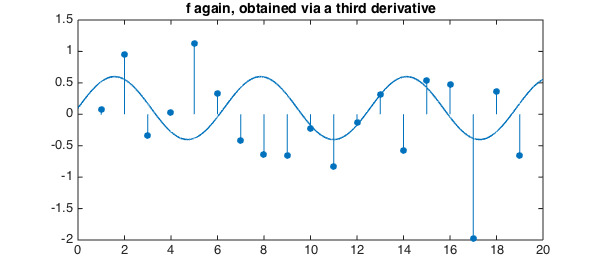Here is a sine wave on the interval $[0,20]$ to which have been added a sequence of Dirac delta functions of random amplitudes, with a constant function then subtracted to make the mean zero:

x = chebfun('x',[0 20]);
f = 0.5*sin(x);
rng(3)
for j = 1:19
f = f + randn*dirac(x-j);
end
f = f - mean(f);
LW = 'linewidth'; lw = 1.6; FS = 'fontsize'; fs = 12;
plot(f,LW,1.6)
title('f:  a sine wave plus a sequence of delta impulses',FS,fs)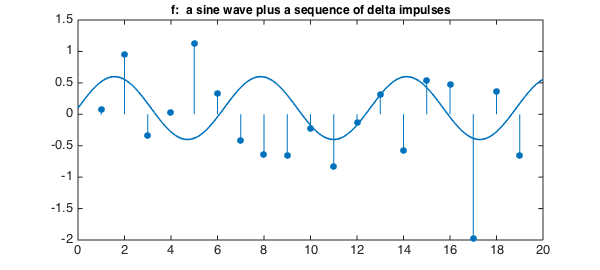Can you explain each of these numbers?

max(f)
ans =
Inf

min(f)
ans =
-Inf

sum(f)
ans =
-4.440892098500626e-16

norm(f,1)
ans =
17.151699883096875

norm(f,2)
ans =
Inf

norm(f,inf)
ans =
Inf


If we integrate $f$ with cumsum, each delta function becomes a jump. The value at the left is $0$ because cumsum always does that, and the value at the right is $0$ because $f$ has zero mean.

g = cumsum(f);
plot(g,'r',LW,1.6)
title('The integral of f',FS,fs)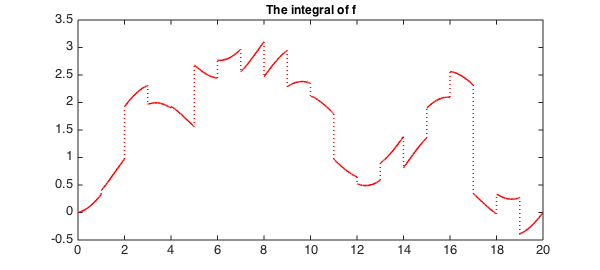If we integrate a second time, we get a continuous function, that is, a function of class $C^0$:

h = cumsum(g);
plot(h,LW,1.6,'color',[0 .7 0])
title('The second integral of f',FS,fs)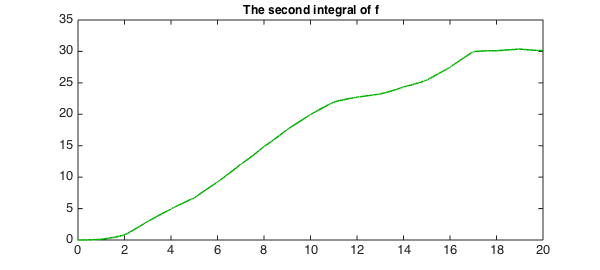Our eye is good at detecting this degree of non-smoothness. One final integration gives a $C^1$ function whose lack of smoothness is not so obvious:

q = cumsum(h);
plot(q,LW,1.6,'color',[1 .5 0])
title('The third integral of f',FS,fs)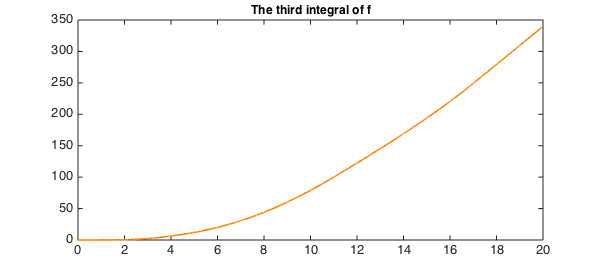Taking the third derivative of this last function brings us back where we started:

f2 = diff(q,3);
plot(f2,LW,1.6)
title('f again, obtained via a third derivative')